# Subtracting decimals

Here you will learn about subtracting decimals, including how to subtract decimals and solve problems that involve the subtraction of decimal numbers.

Students will first learn about subtracting decimals in 5th grade math using concrete models and expand that knowledge into 6th grade using the algorithm for subtraction.

## What is subtracting decimals?

Subtracting decimals is when you subtract numbers involving decimals.

Subtracting decimal numbers is very similar to subtracting multi-digit whole numbers.

Let’s look at strategies for subtracting decimals.

Subtracting decimals with the same decimal places
Using a model

1.66-0.95 = \, ?
● Represent the decimal numbers on the hundredths grid.
● Cross out the squares that are being subtracted.
● Count up the squares that are left and write it as a decimal.

Regrouped 1 to be 10 tenths

Matching 9 tenths and 5 hundredths to be removed.

Subtracting decimals with the same decimal places
Using the algorithm

1.66-0.95 = \, ?
● Stack the numbers with the larger number on top.
● Line up the decimal points and place values.
● Add 0' s as placeholders where necessary.
● Subtract the way you would subtract whole numbers, regroup where
necessary.

The answer in standard form is \bf{0.71}

1.66 rounds to 2 and 0.95 rounds to 1.
The answer should be close to 2-1 = 1.
It is, so 0.71 is reasonable.

Subtracting decimals with different decimal places
Using a model

0.8 - 0.25 = \, ?
● Represent the decimal numbers on the hundredths grid.
● Cross out the squares that are being subtracted.
● Count up the squares that are left and write it as a decimal.
*Each square = \mathit{0.01}

Regrouped 0.80 to be 7 tenths and 10 hundredths

Matching 2 tenths and 5 hundredths to be removed.

Subtracting decimals with different decimal places
Using the algorithm

0.8-0.25 = \, ?
● Stack the numbers with the larger number on top.
● Line up the decimal points and place values.
● Add 0' s as placeholders where necessary.
● Subtract the way you would subtract whole numbers, regroup where
necessary.

The answer in standard form is \bf{0.55}

0.80 rounds to 1 and 0.25 rounds to 0.
The answer should be close to 1-0 = 1.
It is, so 0.55 is reasonable.

Subtracting a decimal from a whole number
Using a model

2-0.85 = \, ?
● Represent the decimal numbers on the hundredths grid.
● Cross out the squares that are being subtracted.
● Count up the squares that are left and write it as a decimal.
*Each square = \mathit{0.01}

Regrouped 1 to be 9 tenths and 10 hundredths

Matching 8 tenths and 5 hundredths to be removed.

Subtracting a decimal from a whole number
Using the algorithm

2-0.85 = \, ?
● Stack the numbers with the larger number on top.
● Line up the decimal points and place values.
● Add 0' s as placeholders where necessary.
● Subtract the way you would subtract whole numbers, regroup where
necessary.

The answer in standard form is \bf{1.15}

1.15 rounds to 1.
The answer should be close to 2-1 = 1.
It is, so 1.15 is reasonable.

### What is subtracting decimals?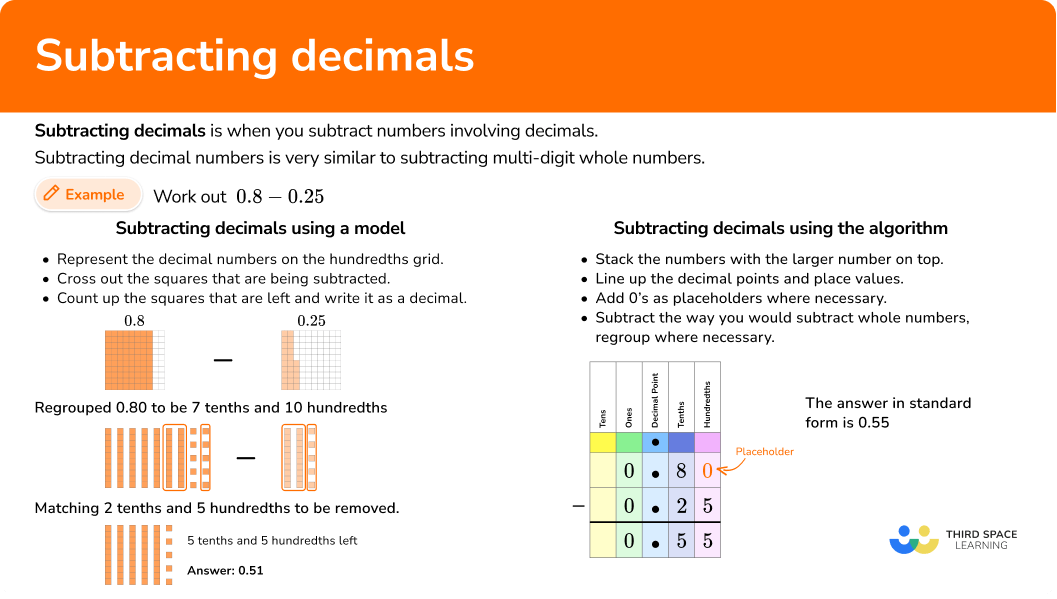## Common Core State Standards

How does this relate to 5th grade math and 6th grade math?

• Grade 5 – Number and Operations – Base Ten (5.NBT.B.7)
Add, subtract, multiply, and divide decimals to hundredths, using concrete models or drawings and strategies based on place value, properties of operations, and/or the relationship between addition and subtraction; relate the strategy to a written method and explain the reasoning used.

• Grade 6 – Number System (6.NS.B.3)
Fluently add, subtract, multiply, and divide multi-digit decimals using the standard algorithm for each operation.

## How to subtract decimals

In order to subtract decimal numbers using models:

1. Show each number using decimal models.
2. Group matching parts.
3. Count up the leftover squares and write the answer in standard form.

In order to subtract decimals:

1. Stack the numbers with the larger one on top, lining up the decimal points and place values.
2. Add \bf{0} ’s as placeholders where necessary.
3. Subtract each column from right to left regrouping when necessary.

## Subtracting decimal examples

### Example 1: subtract decimals using hundredths grid

Subtract 2.03 - 1.2

1. Show each number using decimal models.

2Group matching parts.

3Remove the grouped parts and write what is left.

There are 8 tenths and 3 hundredths left over. The answer in standard form is 0.83.

### Example 2: subtracting numbers with the same amount of decimal places

Subtract the numbers 7.3 - 2.8

All place values lined up.

7.3 rounds to 7 and 2.8 rounds to 3.

The answer should be close to 7 - 3 = 4.

It is, so 4.5 is reasonable.

### Example 3: subtracting decimals with the same amount of decimal places

Subtract the numbers 12.43 - 7.81

Not necessary because the decimals have the same number of decimal places.

12.43 rounds to 12 and 7.81 rounds to 8.

The answer should be close to 12 - 8 = 4.

It is, so 4.62 is reasonable.

### Example 4: subtracting decimals that have different decimal places

Subtract the numbers 0.8 - 0.17

### Example 5: subtracting decimals with different decimal places

Subtract the numbers 9.1 - 3.276

9.1 rounds to 9 and 3.276 rounds to 3.

The answer should be close to 9 - 3 = 6.

It is, so 5.824 is reasonable.

### Example 6: subtracting a decimals where one number is a whole number

Subtract the numbers 5 - 2.081

2.081 rounds to 2.

The answer should be close to 5 - 2 = 3.

It is, so 2.919 is reasonable.

### Example 7: word problem

Micah goes to lunch and buys a burger, fries, and a drink for \$8.49. He gives the cashier a \$20  bill. How much change does he get back?

Micah will get \$11.51 back. Is the answer reasonable? \$11.51 rounds to \$11.50 or \$12.

The answer should be close to  20 - 8.50 = 11.50 or 20 - 8 = 12.

It is, so \$11.51 is reasonable. ### Teaching tips for subtracting decimals • Use visual models to develop students’ understanding of place value along with subtraction. • Although practice worksheets help students master skills, infusing resources that promote conceptual understanding will provide students with deep understanding of the numerical concepts. Strong number sense leads to strong algebraic understanding. • Reinforce estimation strategies so that the decimal numbers make sense to students. ### Our favorite mistakes • Not lining up the decimal points and place values. A common error when subtracting decimals occurs when the decimal points are not lined up but instead the last digits are lined up. • Not placing \bf{0} ’s as placeholders. Subtracting the numbers 10 - 0.51 ### Practice subtracting decimals questions 1. Subtract the numbers 1.04-0.23 1.010.81810.91Stack the numbers with the larger one on top, lining up the decimal points.No 0 ’s are needed as placeholders. Subtract from right to left, regrouping when necessary.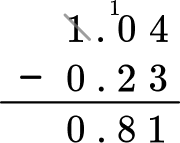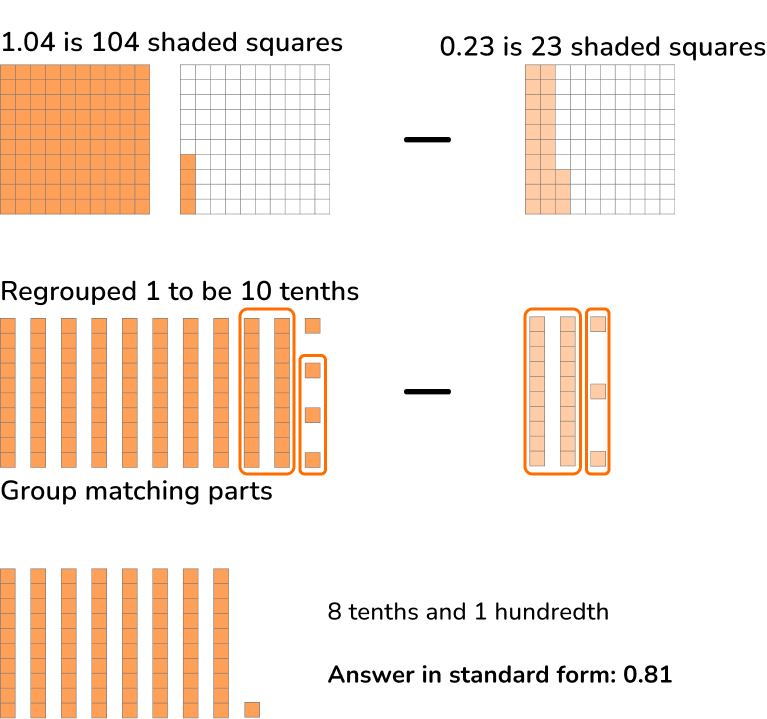Check if the answer is reasonable. 1.04 rounds to 1 and 0.23 rounds to 0. The answer should be close to 1-0 = 1 It is, so 0.81 is reasonable. 2. Subtract the numbers 12.5-7.8 4.75.320.35.7Stack the numbers with the larger one on top, lining up the decimal points.No 0 ’s are needed for placeholders. Subtract from right to left, regrouping when necessary.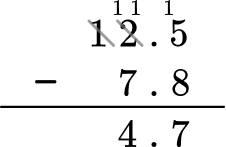The answer in standard form is 4.7 Check to see if the answer is reasonable. 12.5 rounds to 13 and 7.8 rounds to 8. The answer should be close to 13-8 = 5 It is, so 4.7 is reasonable. 3. Subtract the numbers 56.29-2.47 31.593.15953.8254.22Stack the numbers with the larger one on top, lining up the decimal points.No 0 ’s are needed for placeholders. Subtract from right to left, regrouping when necessary.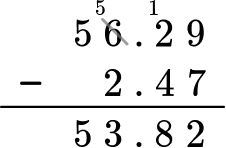The answer is 53.82 Check to see if the answer is reasonable. 56.29 rounds to 56 and 2.47 rounds to 2. The answer should be close to 56-2 = 54 It is, so 53.82 is reasonable. 4. Subtract the numbers 23.4-16.573 7.82716.3397.1736.827Stack the numbers with the larger one on top, lining up the decimal points and place values. Add 0 ’s as placeholders.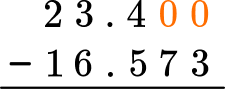Subtract from right to left, regrouping when necessary.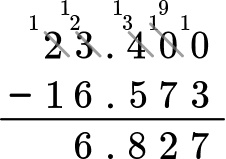The answer is 6.827 Check to see if the answer is reasonable. 23.4 rounds to 23 and 16.573 rounds to 17. The answer should be close to 23-17 = 6 It is, so 6.827 is reasonable. 5. Subtract the numbers 8.29-5.9 2.393.22.43.28Stack the numbers with the larger one on top, lining up the decimal points and place values. Add 0 ’s as placeholders.Subtract from right to left, regrouping when necessary.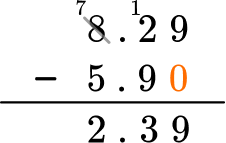Check to see if the answer is reasonable. 8.29 rounds to 8 and 5.9 rounds to 6. The answer should be close to 8-6 = 2 It is, so 2.39 is reasonable. 6. Subtract the numbers 24-11.27 11.0311.3012.3712.73Stack the numbers with the larger one on top, lining up the decimal points and place values. Add 0 ’s as placeholders.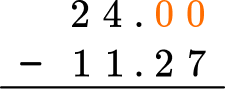Subtract from right to left, regrouping when necessary.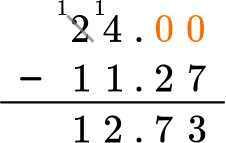Check to see if the answer is reasonable. 11.27 rounds to 11. The answer should be close to 24-11 = 13 It is, so 12.73 is reasonable. 7. Henry and Joseph dumped out all the change in their piggy banks. Henry counted \$6.19 and Joseph counted \$3.46. How much more money does Henry have than Joseph? \$2.73\$9.65\$2.33\$3.73Stack the numbers with the larger one on top, lining up the decimal points and place values. Add 0 s as placeholders.Subtract from right to left, regrouping when necessary.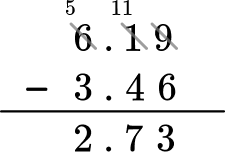Check to see if the answer is reasonable. 6.19 rounds to 6 and 3.46 rounds to 3. The answer should be close 6-3 = 3 It is, so \$2.73 is reasonable.

8. A group of 6 th grade students competed in a race. The table shows their times to the nearest hundredth of a second.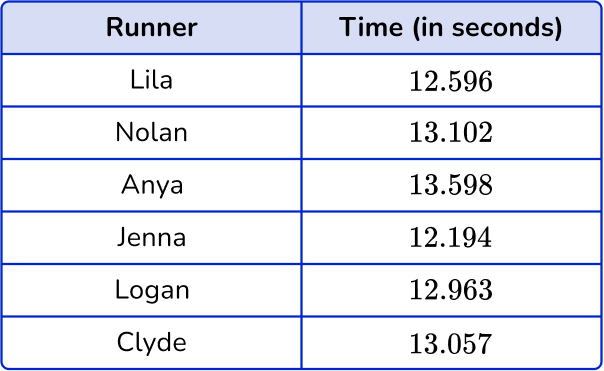How much faster did Jenna run the race than Clyde?

0.943 seconds0.541 seconds0.863 seconds0.769 secondsStack the numbers with the larger one on top, lining up the decimal points and place values.  Add 0 s as placeholders.Subtract from right to left, regrouping when necessary.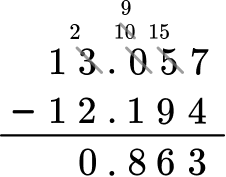Check to see if the answer is reasonable.

13.057 rounds to 13 and 12.194 rounds to 12.

The answer should be close 13-12 = 1

It is, so 0.863 is reasonable.

## Subtracting decimals FAQs

Is subtracting decimals similar to adding decimals?

They have similar procedures, such as lining up decimal points, place values, and adding 0 ’s as place holders. When you subtract decimals in 5th grade and 6th grade it’s important to stack the decimal numbers so that the larger number is on top. When you add decimals, that step isn’t necessary.

Using estimation strategies such as rounding is a good practice when doing decimal operations. Whole numbers are easier to conceptualize than decimal numbers so it’s a good practice to determine if your answer is reasonable.

What decimals should students work with in 5th and 6th grade?

Following the Common Core recommendations, in 5th grade, students should work with tenths place, hundredths place and thousandths place. In 6th grade this is expanded to smaller numbers. Check your state’s specific standards for further clarification.

What are the rules for subtracting decimals?

To subtract decimals, you must stack the numbers with the larger one on top, ensuring that the decimal points and place values are aligned. You may need to add zeros as placeholders in the number with fewer decimal places to maintain alignment. Then, subtract each column from right to left and regroup when necessary. The decimal point must be placed in the same position in the answer as the original numbers.

How do you subtract a larger decimal from a smaller decimal?

To subtract a larger decimal from a smaller decimal, follow the same steps to subtract decimals – align the numbers and decimal points with the larger number on top, use zeros where necessary, then subtract from right to left. This will give you the difference between the two numbers. Your final answer, however, will be negative since the larger decimal is being subtracted from the smaller decimal.

How do you subtract decimals with different decimal places?

To subtract decimals with different decimal places, you will use zeros as placeholders in the number that has fewer decimal places. Then, follow the steps to subtract.

## Still stuck?

At Third Space Learning, we specialize in helping teachers and school leaders to provide personalized math support for more of their students through high-quality, online one-on-one math tutoring delivered by subject experts.

Each week, our tutors support thousands of students who are at risk of not meeting their grade-level expectations, and help accelerate their progress and boost their confidence.